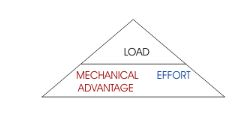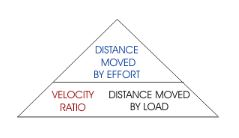Jet Set Go! All about Aeroplanes Jet Set Go! All about Aeroplanes

# Pulley

## What is Pulley?

It is a simple wooden or metallic machine that uses a wheel and rope to lift heavy loads. Nowadays, plastic pulleys are also available in the market to carry small loads. This can be rotated freely about an axis passing through its center. It can change the direction of a force which makes it much easier for people to lift anything. With this, you can pull down on one end to lift the 10 kgs and one-meter high object.## Types of Pulley

• Fixed Pulley

When the block of the pulley is fixed on a high platform, it is known as fixed. An extensible string passes over the groove where its one end is attached to the body to be lifted while the other end is free.

• Movable Pulley

When the block of the pulley is not fixed but carries the load, it is known as Movable. An inextensible string is tied around the groove where its one end is fixed to fixed support while the other end is kept free to apply the effort. As the effort is applied, the block together with the load moves upward.

### Pulley Formula

Following are the formulas that are used when pulleys are used for lifting. There are formulas that are important and they are:

• Mechanical advantage formula: It is defined as the ratio of load to the effort.Using a single formula inside the triangle, mechanical advantage, load, and effort can be generated.

• Velocity ratio: It is defined as the ratio of the distance moved by the effort to the distance moved by the load.Using a single formula inside the triangle, distance moved by the load, velocity ratio, and distance moved by the load can be generated.

• Distance moved by load = (distance moved by effort/Velocity ratio)
• Velocity ratio = (distance moved by effort/Distance moved by load)
• Distance moved by effort = Distance moved by the load*Velocity ratio

#### To know the Concept based on Rotation of Pulley, Watch The Below Video: